# Mini-survey

In the mini-survey of our class about the popularity of individual subjects, it turned out that 11.1% of pupils like mathematics, 18.5% are enjoying languages, 30.4% of pupils like physical education, and the remaining 12 pupils have several popular subjects. How many students are visiting our class?

n =  30

### Step-by-step explanation: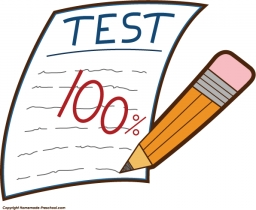Did you find an error or inaccuracy? Feel free to write us. Thank you!Tips to related online calculators
Need help to calculate sum, simplify or multiply fractions? Try our fraction calculator.
Do you have a linear equation or system of equations and looking for its solution? Or do you have a quadratic equation?
Do you want to round the number?

## Related math problems and questions:

• Three subjects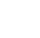In a class of 40 students, 18 passed mathematics, 19 passed accounts, 16 passed economics, 5 mathematics and accounts only, 6 mathematics only, 9 accounts only, 2 accounts and economics only, if each student offered at least one of the subjects. a) how ma
• Jane classWhen asked how many students are in class, Jane said, if we increase the number of students in our class by hundred % and then add half the number of students, we get 100. How many students are in Jane's class?
• Prospering studentsThere are girls and 30 boys in the class. Boys prospering 28, girls all. How many girls are in the class and how many boys are there, if all the prospering students are 95%?
• Alcohol risk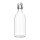The 2009 Youth Risk Behavior Survey found that about 5 out of 12 high school students drank some alcohol in the 30 days preceding the survey. If a high school has 2400 students, how many would be expected to have used alcohol within a 30-day period?
• Marks at schoolThere are 30 students in the class. Five students in the class had a mark three triple at the end of the math certificate, and the other students had a mark of one or two. The average mark in all students' mathematics in the class at the end of the year w
• PupilsThere are 350 girls in the school, and the other 30% of the total number of pupils are boys. How many pupils does the school have?
• StudentsIf the fifth-grade class left 20% of students and in the seventh grade were added two pupils, in the eighth one pupil, the number has not changed in the ninth grade, but it is now tenth students less than it was in the fifth grade. How many pupils are in
• Families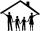In the class, we investigated how many children have families in which they live. How many % of pupils live in families with more than two children? If five families have one child in 9 families, have 2 children, in 5 families have 3 children, in 2 famili
• The bar chartThe bar chart shows the results of the math test. How many% of pupils achieved approximately better results than the average of the class? Diagram: 1-10 pupils, 2-4 pupils, 3-6 pupils, 4-2 pupils, 5-4 pupils. (lowest result are better)
• English and math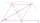1/5 of a class study English, and 3/5 study mathematics. If the class consist of 80 students, how many students study English and mathematics in the class?
• Certificate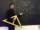There is 31 students in a class. From mathematics was'nt worse mark than 2. Average mark in mathematics was 2. How many students have mark 1 and how many mark 2?
• Ski class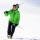Class attend 30 boys and some number of girls. Ski training was attended by 28 boys and all the girls, which was 95% of all students. How many girls attend this class? How many percent is that?
• Lesson exercising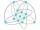The lesson of physical education, pupils are first divided into three groups so that each has the same number. The they redistributed, but into six groups. And again, it was the same number of children in each group. Finally they divided into nine equal g
• In the classroomThere are 30 boys and a few girls in the class. In the six months, 28 boys and all girls benefited, which was 95% of all pupils. How many pupils are there in the classroom?
• Employees1116 people are working in three factory halls. In the first one, there are 18% more than the third, and 60 persons more than the second. How many employees work in individual halls?
• Pizza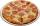A school survey found that 12 out of 15 students like pizza. If 6 students are chosen at random, what is the probability that all 6 students like pizza?
• Unions now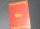The plant has 1,250 employees. The Trade Unions (former ROH, OH) is 18% male, 23% female. Plant management reported that in the trade union is 20% of all employees. How many men and women are in the plant, and how many are men and women in trade unions?